Science Courses / Course / Chapter

# Constructive and Destructive Interference

Kieoshie Alsinan, April Koch
• Author
Kieoshie Alsinan

Kieoshie Alsinan has taught elementary and secondary math and science for 7 years. She has a Master of Science degree in Instruction, Curriculum, and Leadership from The University of Memphis as well as a Bachelor of Science degree in Education from The University of Tennessee at Martin. She also has a Graduate Certificate in Instructional Design and Technology from The University of Memphis. She has an educator's license that permits her to teach all subjects in grades 4 through 8.

• Instructor
April Koch

April teaches high school science and holds a master's degree in education.

Interference occurs when two waves meet. Explore the lesson and examples to learn about the differences between constructive and destructive interference. Updated: 03/22/2022

Show

#### What is an example of destructive interference?

An example of destructive interference is when two sound waves with different frequencies overlap and the noise level or volume decreases.

#### How do you know if it is constructive or destructive interference?

You know if its constructive interference if the resultant wave's amplitude is equal to the sum of the two waves. You know if its destructive interference if the resultant wave no longer disturbs the medium.

#### What is destructive and constructive interference?

Destructive interference is when two waves traveling in the same direction are aligned at the crest of one wave and the trough of the other. The waves cancel out. Constructive interference is when two waves traveling in the same direction overlap, and their crests combine to produce a larger wave.

#### What is an example of constructive interference?

An example of constructive interference is the collision of two sound waves with the same frequency but traveling from two different sources. The sound will get louder.

What happens when two waves meet? Wave interference occurs when two waves meet. Have you ever noticed several speakers in a hall or a music room? One speaker may not always be enough. The speakers are used to increase the loudness of sound in the music room or hall. When soundwaves are produced through all speakers, they travel and can come in contact with each other. This phenomenon is wave interference. Wave interference is defined as an event that occurs when two waves traveling through the same medium collide and create a new wave with different properties. There are two types of wave interference: constructive and destructive interference. They describe what happens when two waves meet. Though both types of interference occur when two waves meet, they produce different results. Constructive interference occurs when two waves collide and combine, but destructive interference happens when two waves collide and cancel out.

To unlock this lesson you must be a Study.com Member.

## Introduction to Interference

I remember when I was in elementary school I used to play jump rope with my friends at recess. We sometimes got bored with actually jumping over the rope and just started making waves with it. Each of us would hold one end of the rope, and we'd both swing our arms up and down, sending giant waves toward each other down the length of the rope. We noticed that the waves would sort of crash into each other, making the rope jump up and down in crazy patterns. Sometimes, though, we'd get the waves going so that they made a perfect pattern that seemed to stand in one place. Standing waves are intriguing phenomena that occur when two waves interfere with each other. To understand why they happen, let's learn more about how interference really works.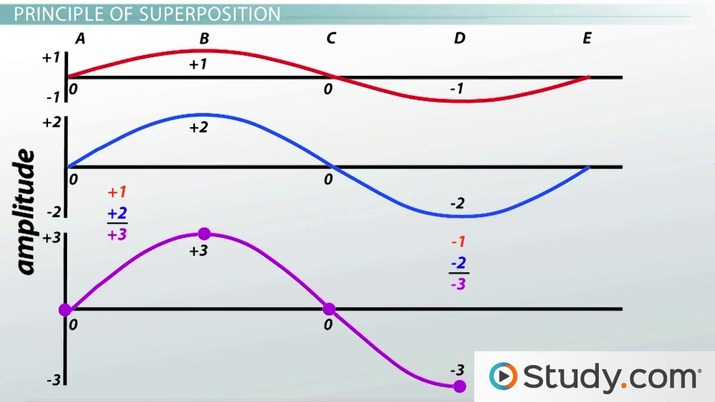An error occurred trying to load this video.

Try refreshing the page, or contact customer support.

Coming up next: The Doppler Effect: Definition, Examples & Applications

### You're on a roll. Keep up the good work!

Replay
Your next lesson will play in 10 seconds
• 0:05 Introduction to Interference
• 0:47 Constructive &…
• 3:00 Principle of Superposition
• 5:43 Standing Waves
• 7:47 Lesson Summary
Save Timeline
Autoplay
Autoplay
Speed Speed

Constructive and destructive waves occur very often in the real world. When sound waves of the same frequency collide, constructive interference may occur. The new sound wave will be twice as loud and have twice the amplitude of the original two waves. As shown in the diagram, two sound waves with a frequency of 1000Hz are traveling towards each other.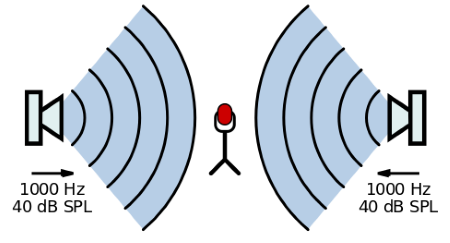The diagram, t=1, models the sound waves hitting the microphone. The crests are represented as positive measurements seen in the colors blue and red. Their amplitudes will overlap and produce a louder sound than the original waves. The amplitude of the new wave will be two times larger.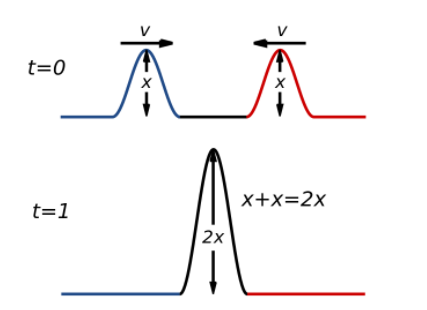Also, sound waves can experience destructive interference. When two sound sources are played, the sound waves travel with different frequencies. The crest of one sound wave will overlap with the trough of the other sound wave, and the sound will cancel out. For this same reason, destructive interference is used in developing noise-canceling headphones.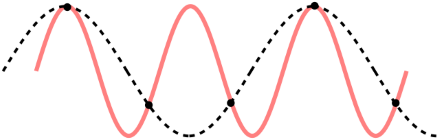The middle of the diagram represents the event in which destructive interference will occur. Imagine this as two sound waves from different sources traveling through a medium. Once they collide, their crest and trough will not match. The crest is represented as a positive measurement, but the trough is represented as a negative number. This difference will result in t=1. Destructive interference will occur, and noise cancellation can be represented as the below flat line in the diagram.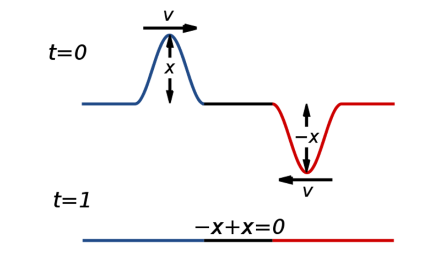To unlock this lesson you must be a Study.com Member.

Constructive interference can be calculated by using the amplitudes of the two overlapping waves.

Constructive Interference = Amplitude of Wave 1 + Amplitude of Wave 2

For example, wave 1 amplitude is 5 meters, and wave 2 amplitude of 7 meters. Then use the equation to find the amplitude of the resultant wave.

5 meters + 7 meters = 12 meters.

Destructive interference = Amplitude of Wave 1 + Amplitude of Wave 2

For example, the amplitude of a trough is -5, and the amplitude of a crest is 6 meters. The amplitude of the resultant wave will equal = -5 meters + 6 meters = 1 meter.

Sometimes the source of the waves is a certain distance apart. As a result, the waves may be wavelengths apart before constructive interference can occur. If the sources of the waves are at the same positions, they will always be a multiple of a wavelength apart. Constructive interference will occur when the two waves meet, although this may not always happen. Whenever the source of each wave is half a wavelength apart or a multiple of a wavelength in addition to half of a wavelength, then destructive interference will occur. In summary, the path difference between the source of each wave determines if the condition of constructive and destructive interference can be met.

The following equation can be used to determine if constructive interference conditions are met:

{eq}R_{1}-R_{2}= 0 + n\Lambda {/eq}

To unlock this lesson you must be a Study.com Member.

Wave interference is the event in which waves make contact. The two types of wave interferences are constructive and destructive interference. Constructive interference occurs when waves two waves with the same frequency while traveling in the same direction collide and produce a wave with a larger amplitude. The amplitude measures the height of a trough or crest of a wave. Destructive interference occurs when two waves overlap at a crest and trough. The crest of one wave cancels out the trough of another wave. The distance between two crests or two troughs is called the wavelength. The Principle of Superposition explains how the amplitude changes due to constructive and destructive interference. It states that the sum of the waves at one point is equal to the displacement of the medium. Therefore, the amplitude of the resultant wave can be found by adding the crests and troughs. When two waves meet, standing waves can be a result as well. Standing waves result from reflection and constructive interference. The original wave is reflected, and the two waves continue to converge and travel with the same frequency only through a contained medium. The following equations can be used to meet the requirements of constructive and destructive interference:

Constructive Interference

• {eq}R_{1}-R_{2}= 0 + n\Lambda {/eq}
• {eq}2x= 0 + n\Lambda {/eq}
• {eq}d sin \Theta =m\Lambda {/eq}

Destructive Interference

• {eq}R_{1}- R_{2}=\Lambda/2 {/eq}
• {eq}R_{1}- R_{2}= \Lambda /2 + n\Lambda {/eq}
• {eq}d sin \Theta =(m + \frac{1}{2})\Lambda {/eq}

To unlock this lesson you must be a Study.com Member.

## Constructive & Destructive Interference

Most of the time, when we think about waves, we tend to imagine a single wave traveling through a medium. When we think about water waves, for example, we imagine one wave traveling through the ocean all by itself, but obviously, that's unrealistic. When is there ever just one wave traveling through the ocean? There are countless waves traveling north and south, east and west. Some ocean waves are bigger and some are smaller. Some waves are caused by the wind, others are caused by cruise ships and others by tons of other things.

Inevitably, some waves are going to cross over or meet with each other. When they do, the reaction between the waves is known as interference. This is the meeting of two or more waves traveling in the same medium. Waves meeting in the same medium actually disrupt each other's displacement. They interfere with each other so that the resulting wave is a completely new and different wave from either one of the original two.

Let's take a look at these two waves: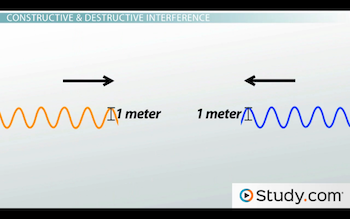They're traveling toward each other in the same medium. One's traveling left and the other's traveling right. They both have the same amplitude of 1 meter. When the two waves meet up, there comes a moment when the crests of both waves end up in the same spot. Their crests overlap, and so their amplitudes add together. Instead of the crest being 1 meter tall, it's 2 meters tall!

When the crests or troughs of two interfering waves meet, their amplitudes add together. This principle is known as constructive interference. So, what happens when the crest of one wave meets the trough of another wave? Well, the opposite happens, and it's called destructive interference. When the crest and trough of two interfering waves meet, one amplitude subtracts from the other.

Let's take our same two waves that we had before. They're still traveling toward each other and they're still 1 meter in amplitude each. But this time, it just so happens that the crest of one wave lines up with the trough of the other wave. Do you know what will happen to the overall amplitude? Well, there won't be any! The crest of the first wave will cancel out the trough of the other wave. The medium experiences zero displacement. The net result is a completely flat surface.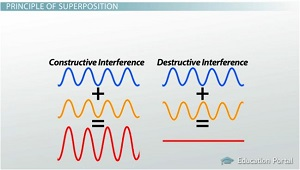## Principle of Superposition

Constructive interference describes a situation where two waves are added together, while in destructive interference, the two waves cancel each other out. But really, the two types of interference are a result of the same thing. When two waves interfere with each other, their displacements at any point are added together to produce the displacement of the medium. Let me show you what I mean.

We'll take two waves that have the same wavelength. One has an amplitude of 1 meter; the other has an amplitude of 2. Let's say they interfere in such a way that all the crests and troughs line up together. So, we'll add up the displacement of both waves at every point, and that will give us the total displacement for the resulting wave when they meet.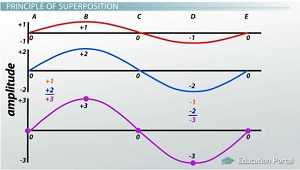At point A, both waves have a displacement of 0. So, the resulting wave is also 0. At point B, we have a plus 1 amplitude for the first wave and a plus 2 amplitude for the second wave. So, the amplitude of the resulting wave would be 3.

Now, at point C, the waves both are back to neutral, and so is the resulting wave. At point D, we add the minus 1 amplitude for the trough of the first wave to the minus 2 amplitude for the trough of the second wave. The result is a sum of minus 3, meaning that our trough in the resulting wave is 3 meters down.

It looks like we got some constructive interference here. Our waves of amplitudes 1 and 2 gave us a resulting wave with a total amplitude of 3.

The exercise we just did illustrates the principle of superposition, the basic rule that tells us how to find the resulting wave from the interference of two different waves. You just treat all the crests as positive numbers and all the troughs as negative numbers. Then, you add up the displacement of the waves for every point. For two interfering waves, the displacement of the medium at any point is the sum of displacements of the waves at that point.

Let's try lining up these waves in a different way. We'll shift our second wave over so that its crest lines up with the trough of the first one. Now, at point A we still have a sum of 0, and the same is true for points C and E. But at point B, the plus 1 for the first wave is countered by the minus 2 of the second wave.

Video Transcript

## Introduction to Interference

I remember when I was in elementary school I used to play jump rope with my friends at recess. We sometimes got bored with actually jumping over the rope and just started making waves with it. Each of us would hold one end of the rope, and we'd both swing our arms up and down, sending giant waves toward each other down the length of the rope. We noticed that the waves would sort of crash into each other, making the rope jump up and down in crazy patterns. Sometimes, though, we'd get the waves going so that they made a perfect pattern that seemed to stand in one place. Standing waves are intriguing phenomena that occur when two waves interfere with each other. To understand why they happen, let's learn more about how interference really works.

## Constructive & Destructive Interference

Most of the time, when we think about waves, we tend to imagine a single wave traveling through a medium. When we think about water waves, for example, we imagine one wave traveling through the ocean all by itself, but obviously, that's unrealistic. When is there ever just one wave traveling through the ocean? There are countless waves traveling north and south, east and west. Some ocean waves are bigger and some are smaller. Some waves are caused by the wind, others are caused by cruise ships and others by tons of other things.

Inevitably, some waves are going to cross over or meet with each other. When they do, the reaction between the waves is known as interference. This is the meeting of two or more waves traveling in the same medium. Waves meeting in the same medium actually disrupt each other's displacement. They interfere with each other so that the resulting wave is a completely new and different wave from either one of the original two.

Let's take a look at these two waves:They're traveling toward each other in the same medium. One's traveling left and the other's traveling right. They both have the same amplitude of 1 meter. When the two waves meet up, there comes a moment when the crests of both waves end up in the same spot. Their crests overlap, and so their amplitudes add together. Instead of the crest being 1 meter tall, it's 2 meters tall!

When the crests or troughs of two interfering waves meet, their amplitudes add together. This principle is known as constructive interference. So, what happens when the crest of one wave meets the trough of another wave? Well, the opposite happens, and it's called destructive interference. When the crest and trough of two interfering waves meet, one amplitude subtracts from the other.

Let's take our same two waves that we had before. They're still traveling toward each other and they're still 1 meter in amplitude each. But this time, it just so happens that the crest of one wave lines up with the trough of the other wave. Do you know what will happen to the overall amplitude? Well, there won't be any! The crest of the first wave will cancel out the trough of the other wave. The medium experiences zero displacement. The net result is a completely flat surface.## Principle of Superposition

Constructive interference describes a situation where two waves are added together, while in destructive interference, the two waves cancel each other out. But really, the two types of interference are a result of the same thing. When two waves interfere with each other, their displacements at any point are added together to produce the displacement of the medium. Let me show you what I mean.

We'll take two waves that have the same wavelength. One has an amplitude of 1 meter; the other has an amplitude of 2. Let's say they interfere in such a way that all the crests and troughs line up together. So, we'll add up the displacement of both waves at every point, and that will give us the total displacement for the resulting wave when they meet.At point A, both waves have a displacement of 0. So, the resulting wave is also 0. At point B, we have a plus 1 amplitude for the first wave and a plus 2 amplitude for the second wave. So, the amplitude of the resulting wave would be 3.

Now, at point C, the waves both are back to neutral, and so is the resulting wave. At point D, we add the minus 1 amplitude for the trough of the first wave to the minus 2 amplitude for the trough of the second wave. The result is a sum of minus 3, meaning that our trough in the resulting wave is 3 meters down.

It looks like we got some constructive interference here. Our waves of amplitudes 1 and 2 gave us a resulting wave with a total amplitude of 3.

The exercise we just did illustrates the principle of superposition, the basic rule that tells us how to find the resulting wave from the interference of two different waves. You just treat all the crests as positive numbers and all the troughs as negative numbers. Then, you add up the displacement of the waves for every point. For two interfering waves, the displacement of the medium at any point is the sum of displacements of the waves at that point.

Let's try lining up these waves in a different way. We'll shift our second wave over so that its crest lines up with the trough of the first one. Now, at point A we still have a sum of 0, and the same is true for points C and E. But at point B, the plus 1 for the first wave is countered by the minus 2 of the second wave.

To unlock this lesson you must be a Study.com Member.

### Register to view this lesson

Are you a student or a teacher?

Back

### Resources created by teachers for teachers

Over 30,000 video lessons & teaching resources‐all in one place.Video lessonsQuizzes & WorksheetsClassroom IntegrationLesson Plans

I would definitely recommend Study.com to my colleagues. It’s like a teacher waved a magic wand and did the work for me. I feel like it’s a lifeline.

Jennifer B.
TeacherCreate an account to start this course today
Used by over 30 million students worldwide